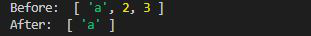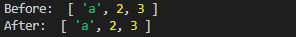Open In App
Related Articles
• Write an Interview Experience
• Lodash
• Lodash Introduction

# Lodash _.difference() Function

The _.difference() function is used to remove a single element or the array of elements from the original array. This function works pretty much same as the core function of JavaScript i.e filter.

Syntax :

`_.difference(array, [values]);`

Parameters: This function accept two parameters as mentioned above and described below:

• array: It is the array from which different elements are to be removed.
• values: It is the array of values that are to be removed from the original array.

Note:

• We can use single value or the array of values. But if only single Integer is given then it will not effect the original array.
• Please install the library before going further using npm install lodash.

Below examples illustrate the _.difference() function in Lodash:

Example 1: When array of values is given.

## Javascript

 `// Requiring the lodash library``let lodash = require(``"lodash"``);` `// Original array``let array = [``"a"``, 2, 3];` `// Values to be removed from``// the original array``let values = [2, 3]``let newArray = lodash.difference(array, values);``console.log(``"Before: "``, array);` `// Printing array``console.log(``"After: "``, newArray);`

Output:Example 2: When an empty array is given, there will be no change in the origin a array.

## Javascript

 `// Requiring the lodash library``let lodash = require(``"lodash"``);` `// Original array``let array = [``"a"``, 2, 3];` `// Values to be removed from``// the original array``let values = []``let newArray = lodash.difference(array, values);``console.log(``"Before: "``, array);` `// Printing array``console.log(``"After: "``, newArray);`

Output:Note: This function returns the original array if the value array is single value, empty array or object of arrays.